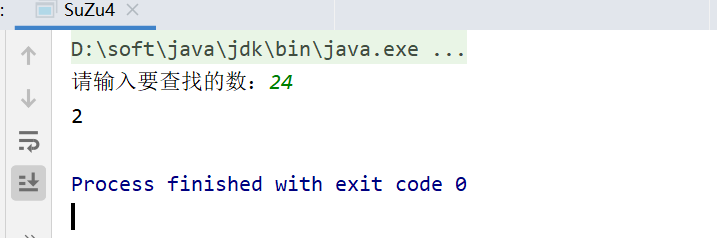• 2020-04-26 23:05:16

前提是数组已经排好序，（查18的下标）
数值 11 12 13 14 15 16 17 18 19 20
下标 0 1 2 3 4 5 6 7 8 9
 第一步，首先取得数组 0~9 的中间元素中间元素的位置为：（开始下标 0 + 结束下标 9）/2=下标 4通过下标 4 取得对应的值 15
18 大于 15，那么我们在后半部分查找
 第二步，取数组 4~9 的中间元素
4~9 的中间元素=（下标 4 + 1 +下标 9）/2=下标 7
下标 7 的值为 18，查找完毕，将下标 7 返回即可

对于有重复元素的有序数组，二分查找需要注意以下要点：
if (val <= A[m]) h = m;
因为 h 的赋值为 m 而不是 m - 1，因此 while 循环的条件也就为 l < h。（如果是 m - 1 循环条件
为 l <= h）

1. public int getPos(int[] A, int n, int val) {
2. int l = 0, h = n - 1;
3. while (l < h) {
4.   int m = l + (h - l) / 2;

5.   if (val <= A[m]) h = m;

6.   else l = m + 1;

7. }
8. return A[h] == val ? h : -1;
9. }算法 二分法
更多相关内容
• 所谓的二分查找，指的是将待查的数据序列而分化，然后对比中间中间值和要查找值，判断结果，相等则找到，小于则在左边的子序列找，大于则在右边的子序列找
• java折半查找

折半查找操作：使用折半查找有序数组中元素。找到返回索引（在数组中的位置），不存在输出-1。
分析：折半查找的前提是数组有序。
假如有一组数为3，12，24，36，55，68，75，88要查给定的值24.
可设三个变量front，mid，end分别指向数据的上界，中间和下界，mid=（front+end）/2.
（1)开始令front=0（指向3），end=7（指向88），则mid=3（指向36）。因为mid>x，故应在前半段中查找。
（2)令新的end=mid-1=2，而front=0不变，则新的mid=1。此时x>mid，故确定应在后半段中查找。
（3)令新的front=mid+1=2，而end=2不变，则新mid=2，此时a[mid]=x，查找成功。
（4）如要查找的数不是数列中的数，例如x=25，当第三次判断时，x>a[mid]，按以上规律，令front=mid+1，即front=3，出现front>end的情况，表示查找不成功。

import java.util.Scanner;
public class SuZu4 {
public static void main(String[] args) {
Scanner sc=new Scanner(System.in);
int[] a = {3, 12, 24, 36, 55, 68, 75, 88};
System.out.print("请输入要查找的数：");
int n =sc.nextInt();

int front = 0;
int end = a.length - 1;
int mid = (front + end) / 2;
do {
int jg;
if (a[mid] > n) {
end = mid - 1;
mid = (front + end) / 2;
if (end < front) {
System.out.println("查找不成功");
return;
}
} else if (n > a[mid]) {
front = mid + 1;
mid = (front + end) / 2;
if (front > end) {
System.out.println("查找不成功");
return;
}
}
} while (a[mid] != n);
System.out.println(mid);
}
}### 折半查找递归操作


import java.util.Scanner;

public class SuZu62 {
public static void main(String[] args) {
int[] a = {3, 12, 24, 36, 55, 68, 75, 88};
Scanner sc = new Scanner(System.in);
System.out.print("请输入要查找的数：");
int n = sc.nextInt();
int s = seek(a, 0, a.length - 1, n);
System.out.println(s);

}

public static int seek(int[] arr, int front, int end, int n) {
int mid = (end + front) / 2;
if (front <= end) {
if (arr[mid] == n) {
return mid;
} else if (arr[mid] > n) {
return seek(arr, front, mid - 1, n);
} else {
return seek(arr, mid + 1, end, n);
}
} else {
return -1;
}
}
}

结果如上

展开全文开发语言 后端
• 哈喽，很高兴见到大家，这次是用java语言...public class 折半法查找 { public static void main(String[] args) { // TODO 自动生成的方法存根 Scanner reader=new Scanner(System.in); int n=0; System.ou

哈喽，很高兴见到大家，这次是用java语言写的折半查找法，实际上，
小白通过对比C语言里的二分法，小白发现，这两种办法的原理相同。
可以参考高中学的查找零点的办法。

import java.util.Scanner;
public class 折半法查找 {

public static void main(String[] args) {
// TODO 自动生成的方法存根
int n=0;
System.out.println("请输入数组的长度：");
int a[]=new int[n];
int i=0,j=0,t=0;
System.out.println("输入数组元素：");
for(i=0;i<n;i++){				/*这一部分是输入数组元素*/
}
for(i=0;i<n-1;i++){				/*这一部分是冒泡排序*/
for(j=0;j<n-i-1;j++){
if(a[j]>a[j+1]){
t=a[j+1];
a[j+1]=a[j];
a[j]=t;
}
}
}
System.out.println("请输入要查找的数：");
int mid=0,low=0,high=n;		/*low是数组的左下标，high是数组的右下标，mid是它们的中间值*/
mid=(high+low)/2;
while(x!=a[mid]) {
if(x<a[mid]) {
high=mid-1;
mid=(low+high)/2;
}
else if(x>a[mid]) {
low=mid+1;
mid=(low+high)/2;
}
if(low==mid)
break;
}
if(x==a[mid]) {
System.out.println("存在该数："+x+"该数在数组里的位置："+mid);
}
else if(x==a[high]) {
System.out.println("存在该数："+x+"该数在数组里的位置："+high);
}
else
System.out.println("数组里不存在该数");

}

}


来看一下下面这个例子小白很希望大家留言，期待下次见面，再见。

展开全文算法
• 折半查找是效率较高的一种查找方法。假设有已经按照从小到大的顺序排列好的五个整数a0~a4，要查找的数是X，其基本思想是： 设查找数据的范围下限为l=1，上限为h=5，求中点m=(l+h)/2，用X与中点元素am比较，若X等于...

折半查找法是效率较高的一种查找方法。假设有已经按照从小到大的顺序排列好的五个整数a0~a4，要查找的数是X，其基本思想是： 设查找数据的范围下限为l=1，上限为h=5，求中点m=(l+h)/2，用X与中点元素am比较，若X等于am，即找到，停止查找；否则，若X大于am，替换下限l=m+1，到下半段继续查找；若X小于am，换上限h=m-1，到上半段继续查找；如此重复前面的过程直到找到或者l>h为止。如果l>h，说明没有此数，打印找不到信息，程序结束。

方法一：

package suanfa;

import java.util.*;

public class zheban {

public static void main(String[]args)

{

int[]a={1,3,6,8,9,89,765};

int time=search(a,8);

System.out.println(time);

}

public static int search(int[]a,int key)

{

int low=1;

int high=a.length;

int mid;

while(low

{

mid=(low+high)/2;

if(key==a[mid-1])

return mid-1;

else if(key>a[mid-1])

low=mid+1;

else

high=mid-1;

}

return 0;

}

}

方法二：

package m;

import java.util.*;

public class zheban {

public static int[]data={1,3,5,7,9,11,22,44,67,89};

public static void main(String[]args)

{

System.out.println("please enter the data you will find:");

Scanner scan=new Scanner(System.in);

int key=scan.nextInt();

zheban(key,0,9);

}

public static boolean zheban(int key,int low,int high)

{

int l=low;

int h=high;

int mid;

while(l

{

mid=(l+h)/2;

if(data[mid]==key)

{

System.out.println("find "+"the data is in array "+(mid+1));

return true;

}

else if(key

h=mid-1;

else if(key>data[mid])

l=mid+1;

}

System.out.println("failed");

return false;

}

}

展开全文• java 折半查找(二分查找)实例，需要的朋友可以参考一下
• } //折半的第二种方式 public static int halfSerach_2(int[] arr,int key){ int min = 0,max = arr.length-1,mid; while(min){ mid = (max+min)>>1; if (key>arr[mid]) { min = mid+ 1; }else if(keyarr[mid]) { ...
• 在数组中用java折半法查找指定的数字，提供了2个方法，一个是递归另一个不是递归方法，好东西大家分享。。。
• 折半插入排序，又称二分插入排序，是直接插入排序的改良版，也需要执行i-1趟插入，不同之处在于，第i趟插入，先找出第i+1个元素应该插入的的位置，假定前i个数据是已经处于有序状态。
• 折半查找是效率较高的一种查找方法。假设有已经按照从小到大的顺序排列好的五个整数a0~a4，要查找的数是X， 其基本思想是： 设查找数据的范围下限为l=0，上限为h=4，求中点m=（l+h）/2，用X与中点元素am比较，若X...
• 折半查找介绍：折半查找，找的是一个有序列表。理论就是先找中间的，如果中间数大于大于目标数，那么就只需要找上半份数据中再折半查找就可以了。一直到找到为止，不用遍历所有数据，效率很高。实现折半查找的方法...
• 首先，Java折半查找适用于有序数组中某一元素下标的查找。例如： //折半查找 public class halfSerach { public static void main(String[] args) { int[] a = {12,23,34,36,47,108}; int index = ...
• // 顺序折半查找下标 public static int BinarySearch(int key, int[] num_list) { int smaller = 0; int bigger = num_list.length - 1; while(smaller <= bigger){ int mid = smaller + (bigger -...list 算法 python
• /* 折半插入排序 / 折半插入排序是对直接插入排序的改进，对于直接...import java.util.Arrays; public class BinaryInsertSort { public static void main(String[] args) { int[] data = new int[] {9, -16, 29数据结构
• 先用***选择排序数组***， 对于从小到大排序的数组，只要判断数据是否和数组中间的值相等， 如果不相等， 当该数据小于中间元素的值时，就在数组的前一半数据中继续***折半***寻找； 当该数据大于中间元素的值...
• while (arr[mid]!=key) //当选定要比较的下标值与折半下标不相同时{if (key>arr[mid]) //如果选定下标大于折半下标min=mid+1; //最小下标移动到折半下标的下一下标处else if (ke...
• high 时表示查找区间为空,查找失败 } Java 代码: /** * 二分查找算法 * * @param srcArray 有序数组 * @param target 被查找的元素 * @retur......6 、不断利用循环和折半查找算法查找一个整数 n 是否在一个无序的 ...
• 二分查找算法思想：又叫折半查找，要求待查找的序列有序。每次取中间位置的值与待查关键字比较，如果中间位置的值比待查关键字大，则在前半部分循环这个查找的过程，如果中间位置的值比待查关键字小，则在后半部分...
• 下面的代码中展示有简单查找和二分查找（也叫折半查找），将进行依次分析。 /* 需求：查找某个元素在数组中的位置 思路： 遍历数组中的每个元素与所要查找的元素进行比较，相同时返回其索引值 步骤： 1.定义...算法
• Java 算法之折半查找 算法介绍 折半查找要求线性表是有序的，即表中记录按关键字有序（假设是递增有序的）。 折半查找的基本思路：设 R[low, ···, high] 是当前的查找区间，首先确定该区间的中间位置 mid = ...数据结构 算法
• JAVA实现折半查找算法 package demo; import java.util.Scanner; public class wdnmd2 { public static void main(String[] args) { int[] arr = {10, 20, 30, 40, 50, 60}; Scanner sc = new Scanner(System....算法
• // 折半查找（二分查找） /*--先从数组中间开始找，如果查找的数>中间值，则在后面找；如果查找的数<中间值，则在前面面找 --如果第一次找不到，则二分从后面（前面）的中间继续找，直到起始位置>...
• 算法实现(java): [code lang=”java”] public class ErFenTest { public static int recursionBinarySearch(int[] arr,int key,int low,int high){ if(key [low] || key > arr[high] || low > high){ return -1;...
• java 快速排序 折半查找的界面实现 （递归与分治
• (2)若目标元素>mid所对应的元素值，则high不变，low=mid+1 若目标元素 (3)重复第二个步骤，注意low不可能大于high，如果该元素存在，最终有low=high=mid，反之则元素不存在 import java.util.Scanner; public class...二分查找 数据结构
• public class ArraySearch { public static void main(String[] args) { // 数组元素第二种查找方法(二分查找/折半查找) int[] 数组名={2,6,8,9,100}; Scanner sc=new Scanner(System.in); System.out.print(...算法
• JAVA基础之二分查找 简介 二分查找又称折半查找,优点是比较次数少查找速度快,平均性能好,占用系统内存较少; 缺点是要求待查表为有序表,且插入删除困难. 因此折半查找方法适用于不经常变动而查找频繁的有序列表...二分查找
• // 折半查找 public static void main(String[] args) { int[] arr = {2,6,3,1,8,9,10}; int index = binarySearch(arr,8); System.out.print(index);//输出 4 } /** * * @param arr 要进行查找的数组......

# java折半法java 订阅